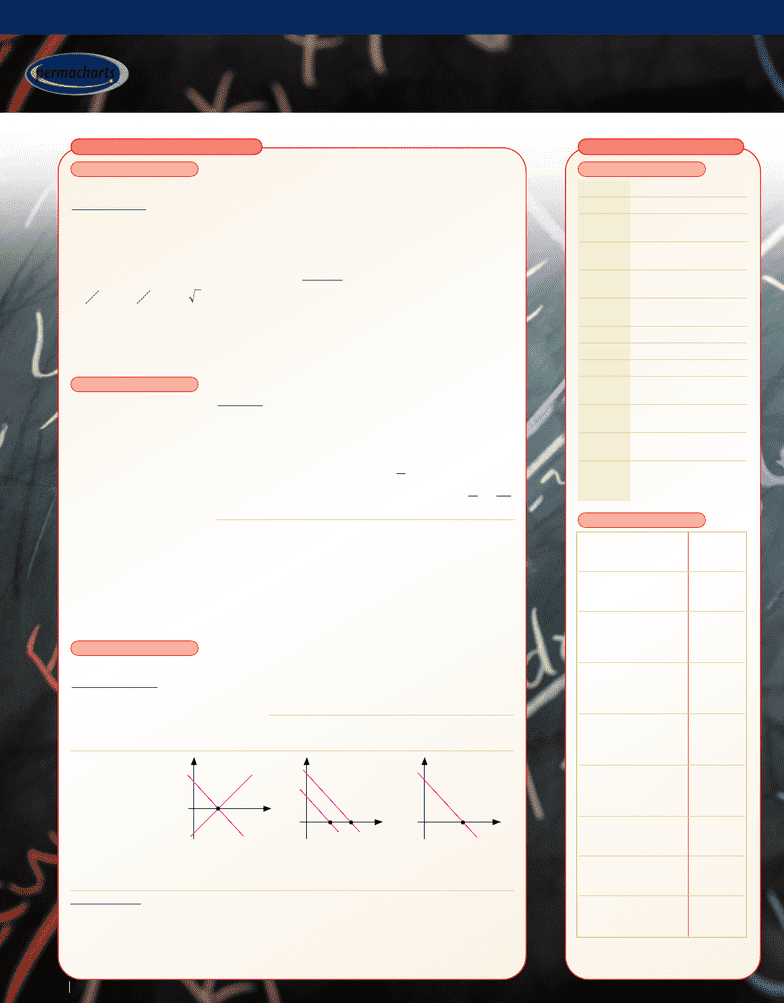Get 2 days of unlimited access
Class Notes (1,000,000)
US (420,000)
UNE (30)
(3)
All (2)
Reference Guide

# Intermediate Algebra - Reference Guides

Department
MATH
Course Code
MATH 504
Professor
All
Chapter
Permachart

This preview shows page 1. to view the full 4 pages of the document.LINEAR EQUATIONS INEQUALITIES
l e a r n r e f e r e n c e r e v i e w
Intermediate Algebra
Intermediate Algebra
23
3211
324 1
323
3211
01110 32
223
07519
01110 32
23
0
32
21311
72
xyz
xyz
xyz
RR xyz
xyz
xy z
RR xyz
xyz
xy z
RR xyz
x
−+=
+−=
−+=
−+=
+−=
−+ =
−+=
+−=
−+ =
+
−+=
+77519
00
123
15
7
15
7
yz
xy z
zyx
−=
++ =
=− = =
34334
43 43
9510 8 0213 4
334
02135
0213 4
34
0213
31
21 32
xyz R R xyz
xy z xy z
xy z xy z
RR xyz
xy z
xy z
RR xyz
xy
+−= − +−=
++ = ++ =
++ = ++ =
+−=
++ =
++ =
+−=
++zz
xyz
=
++=
5
000 9
Zero term(s) on diagonal: No solution
Word Problem
• Two sums are to be invested in
parts yielding 4% and 5% return:
\$40,000 to yield a total return of
\$1800 and \$12,000 to yield \$200
• How should the investment be split?
ONE UNKNOWN
Word Problems
The sum of two numbers is 12 and one
number is three times the other
• What are the numbers?
x+ 3x= 12 4x= 12 x= 3
By how much should the radius of a circle
(r= lm) be increased to double the area?
Salt crystallizes from water when its
concentration reaches 50% How much
water should be evaporated from 40 kg of
a 20% solution to trigger crystallization?
0.5(40 – x) = 0.2 x 40 x= 24 kg
Two trains traveling at an average speed of
120 and 80 km/hr leave at a distance
300 km apart When will they meet?
120t+ 80t= 300 t= 1.5 hrs
Example
2(y + 3) = 5(y – 1) 7(y – 3) 4y = 10
2y + 6 = 5y – 5 7y + 21 y = 10/4 = 5/2
NUNKNOWNS
• Linear equations in n
unknowns can be solved
by Gaussian Elimination,
where the constant
coefficients of the
equations are arranged in
an array
• Various multiples of the
rows are added to or
subtracted from other
rows until zeros are
obtained above or below
the diagonal of the
coefficients
• The unknowns are then
calculated by successive
back-substitutions; if one
or more of the diagonal
elements is zero, then no
unique solution exists
• Linear equations in one unknown have the form ax + b = 0with the solution x = – b/a
TWO UNKNOWNS
• Two linear equations in two unknowns have the form: a1x+ b1y= c1a2x + b2y= c2
Solution Methods
By substitution: Solve for one unknown
(xor y) in either of the equations and
substitute in the other equation
24 24 1
23 348 2
xy y x x
xy x x y
−= =− =
+= =+ =
25 25 107
274 22574
xy y x
yx x x
−= =− −=
=+ − =+( ) no solution
xy=1
x+y=1
x
y
x+y=2
x
y
12
x+y=1
x+y=1
x
y
1
5x+5y=5
Consistent equations
have one solution:
y= 0, x= 1
Inconsistent equations
have no solution as
lines are parallel
Dependent equations
have an infinite
number of solutions
x + y = 40 000
0.05x + 0.04y = 1 800
x = 20 000
y = 20 000
x + y = 12 000
.05x + 0.4y = 200
x = –28 000
Therefore, no solution
Examples
By graphing: Plot
both equations
yielding two straight
lines • The point of
intersection represents
the solution If there
is no intersection,
then the solution does
not exist or there is
an infinite number of
solutions
ππ
() .121 2 1 0414
22
+= ⇒==xxm
DEFINITIONS
RULES OF OPERATION
x+y=1
xy=1 x+y=1
x+y=2 x+y=1
5x–5y=5
a > b ais greater than b
a < b ais smaller than b
a b ais greater than or
equal to b
a b ais less than or equal
to b
a > b, Inequalities having the
c > d same sense
b < a < c ais greater than b and
less than c
a <> b ais not equal to b
a > 0 ais a positive number
a < 0 ais a negative number
ıaı awithout sign =
absolute value of a
ıaı 0 ıaı is always greater
than or equal to zero
a > b, Inequalities having the
c < d opposite sense
b a c ais greater than or
equal to b and less
than or equal to c
Addition of the No
same constant change
on both sides
Subtraction of the No
same constant change
from both sides
Multiplication/ No
division of both change
sides by a positive
constant
Multiplication/ Inequality
division of both sign
sides by a negative reversed
constant
Raising each side No
(assumed positive) change
to a positive
number
Raising each side Inequality
(assumed positive) sign
to a negative reversed
number
inequalities of the change
same sense
Transfer of a term Term
to the other side changes
sign
Removal of –b <a<b
absolute sign
from |a| < b
TM
permacharts
© 1998-2012 Mindsource Technologies Inc.
INTERMEDIATE ALGEBRA • A-631-91www.permacharts.com
###### You're Reading a Preview

Unlock to view full version